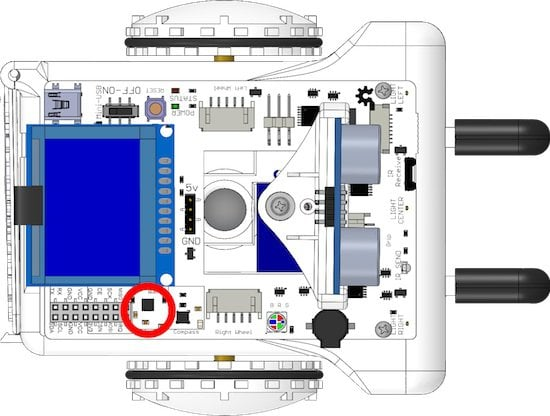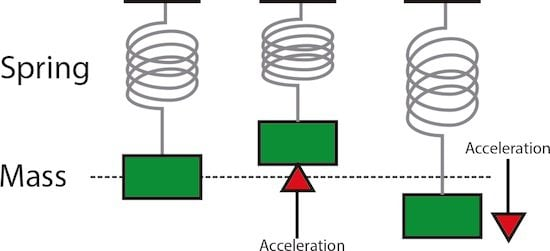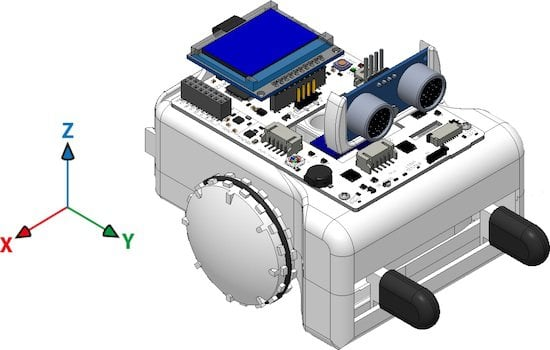# Accelerometer

SparkiDuino
Easy

### Lessons You Should Know

Sparki has a 3-Axis accelerometer. It is used to detect the acceleration Sparki is experiencing. Usually, this mostly means the force of gravity. It can do this in all 3 XYZ axis, or directions, which are left/right (X), forward/backwards (Y), and up/down (Z). This is the same part that smartphones use to tell how you’re tilting them.## What is Acceleration?

Acceleration is how fast something speeds up. If we took a super turbo-charged Sparki and had it going 10 meters per second (36kph, 22 mph), the velocity is 10 meters per second, written as 10 m/s. If the car is reaching 10 meters per second in 10 seconds, you can say the acceleration is 1 meters per second per second, or 1 meters per second squared, written as 1 m/s2.Gravity is also a force that causes acceleration. Objects that are falling will fall faster and faster with acceleration.The only thing that keeps most things from falling is that they are being pushed up by the ground. Gravity is the acceleration that Sparki measures when it is sitting flat on a surface.

## How It Works

The accelerometer is what’s called a microelectromechanical, or MEMs device. MEMs are made of very tiny mechanical parts and wires too small to see, made in the same way microchips are made. Inside of the part, too tiny to see, are tiny springs. When the part is accelerated, these springs compress or relax. By measuring the amount these springs compress or relax, the part is able to measure the acceleration it is experiencing.The part has 3 different axis, or directions, of these spring-masses. They are left/right (X), forward/backwards (Y), and up/down (Z):The direction of the arrows shows the positive direction of the reading. If the acceleration is in the opposite direction, the reading will be negative. For example, if Sparki is sitting flat on a level table, the Z axis reading should be -9.8 m/s2. If Sparki is then flipped upside-down, the reading would be positive 9.8 m/s2.

## Using the Part

With the basic Sparki code in place, you can measure the acceleration in each axis using these commands: This command returns the acceleration the accelerometer reads in meters per second squared ( m/s2), in the form of a float. For example, if Sparki is sitting flat on a level table, sparki.accelZ();  should read -9.8 m/s2. For conversion sake, 1G = 32 ft/s2 = 9.8 m/s2. This is also known as 1G, or one unit of earth’s gravity SparkiArduino already has code examples for you to use: File > Examples > Accelerometer